# 0273685988_ch03.ppt

Mar. 27, 2023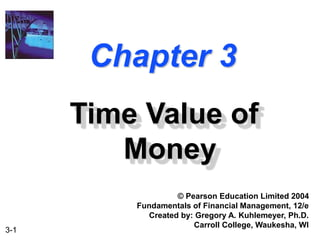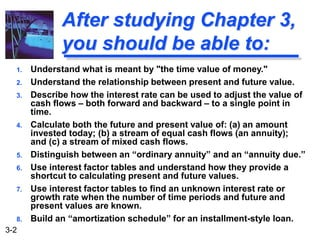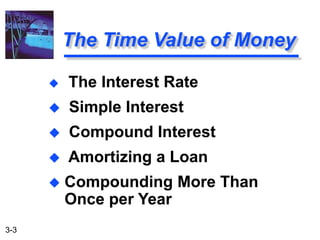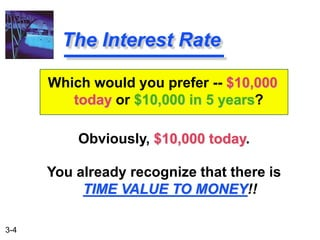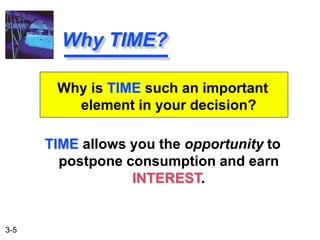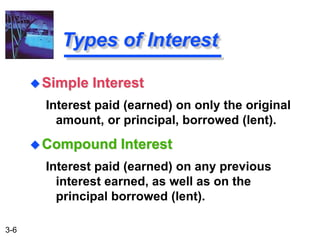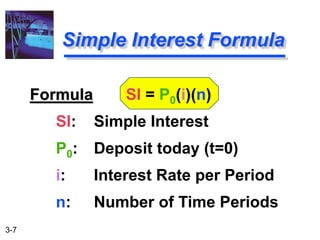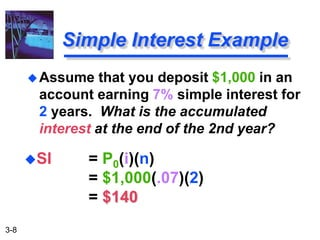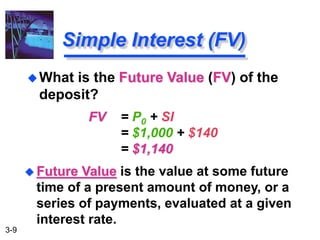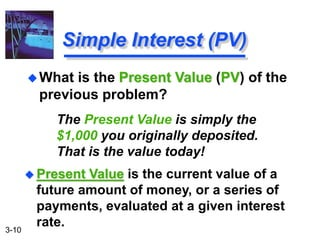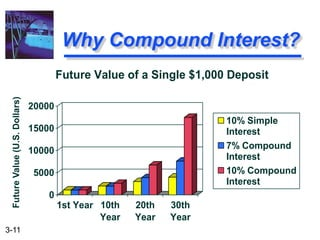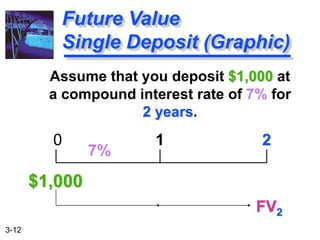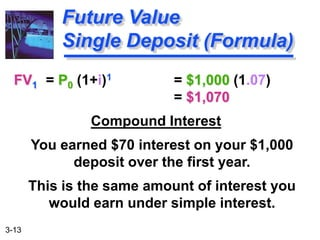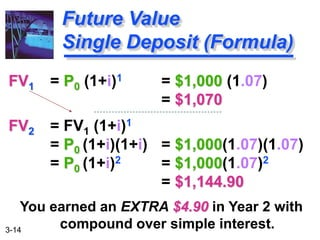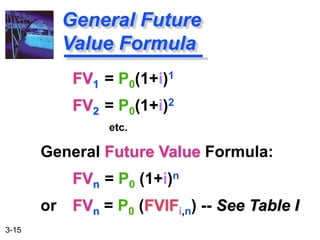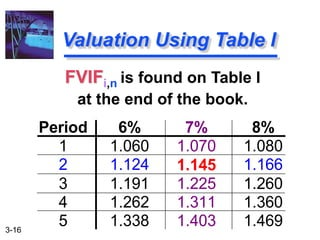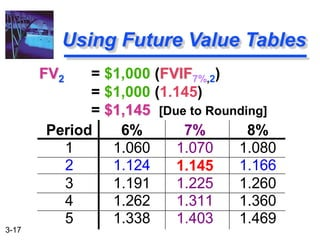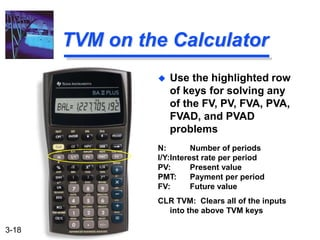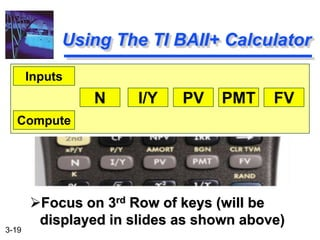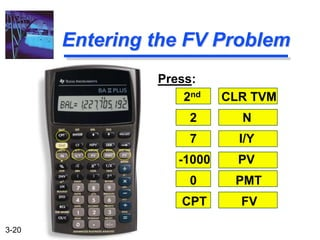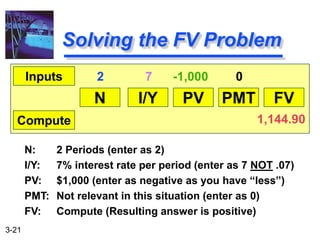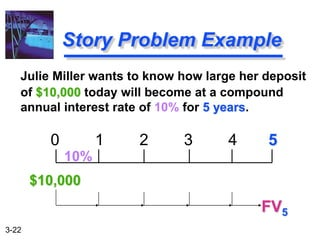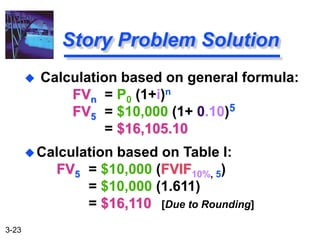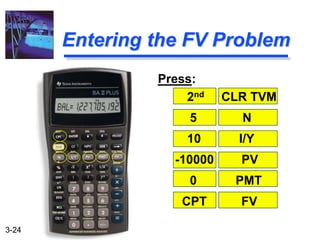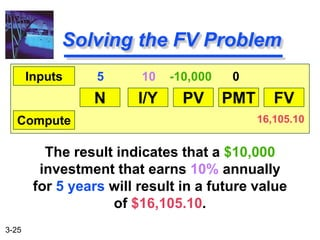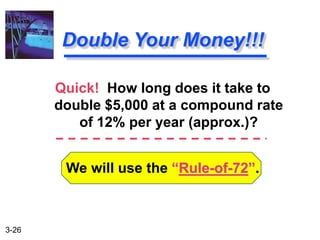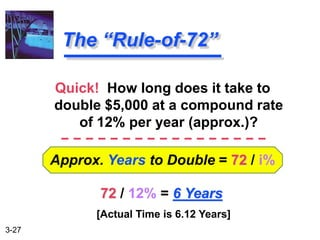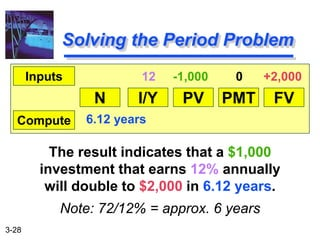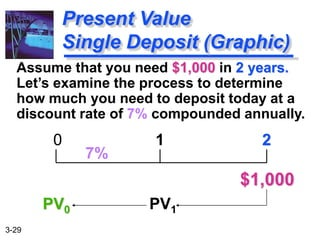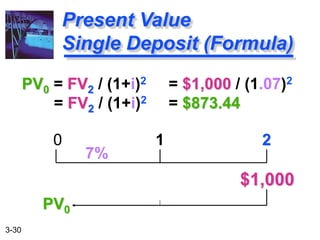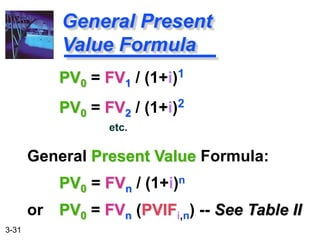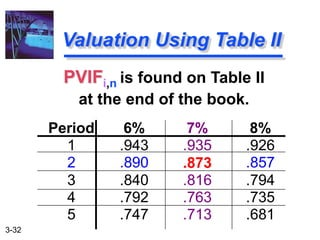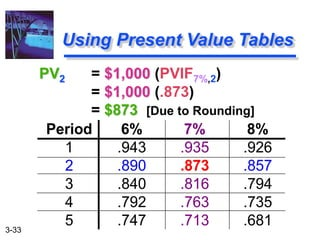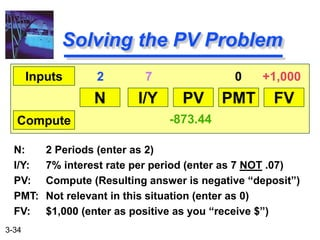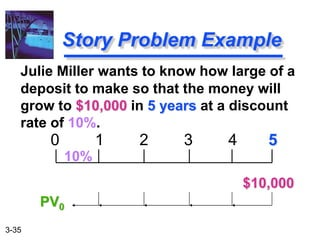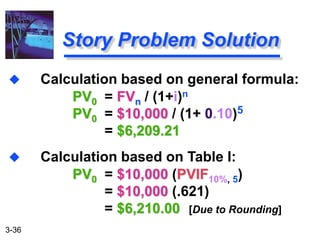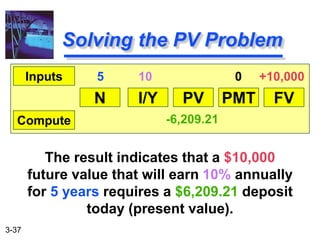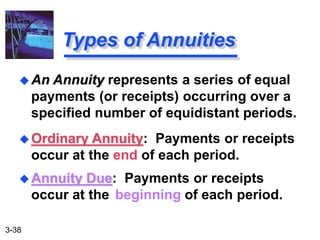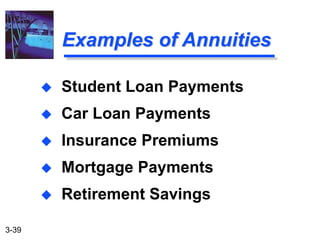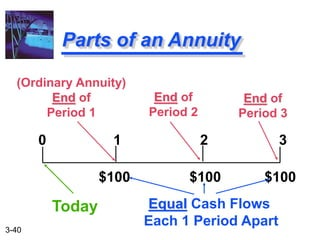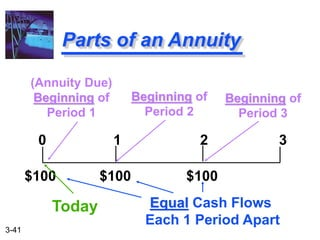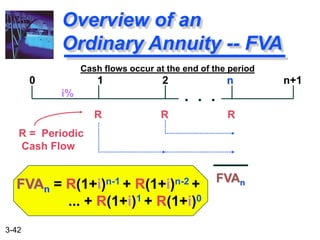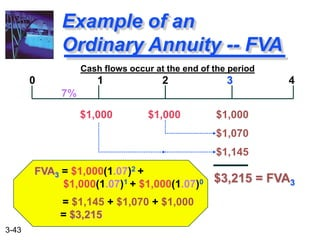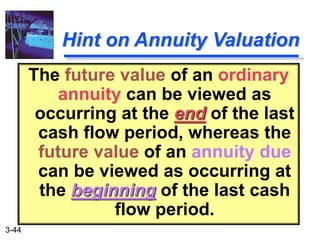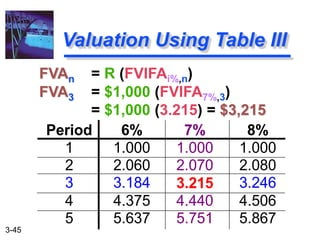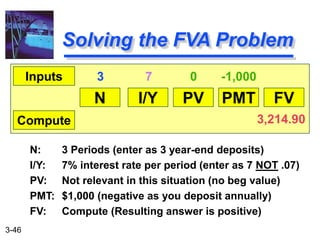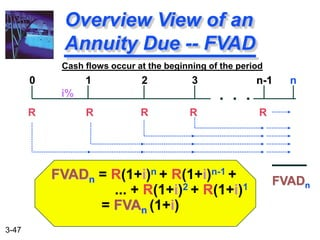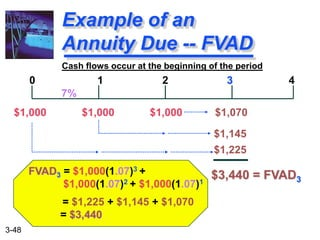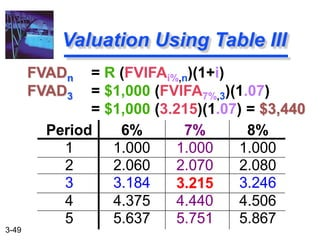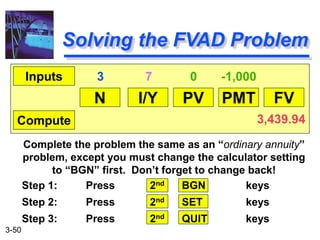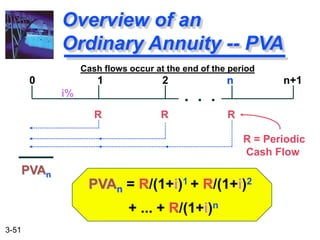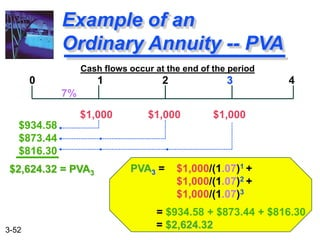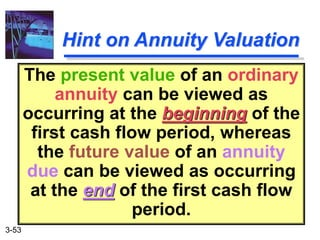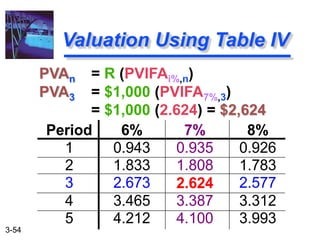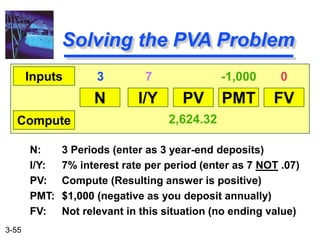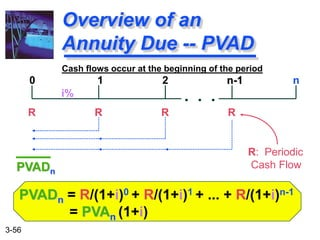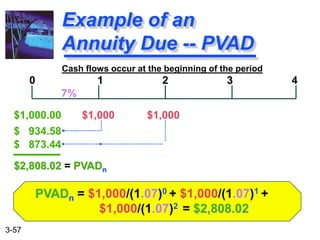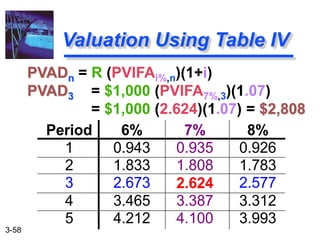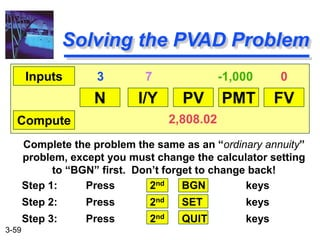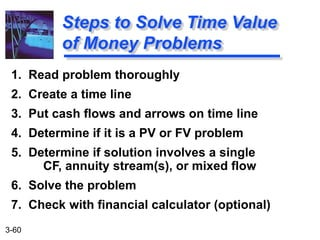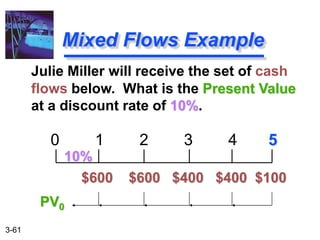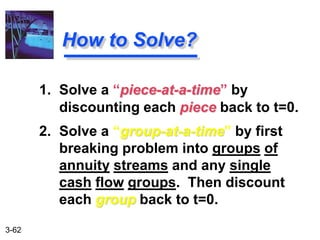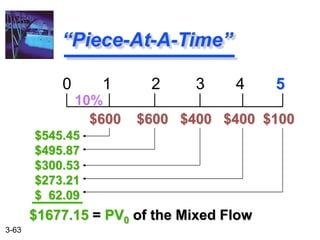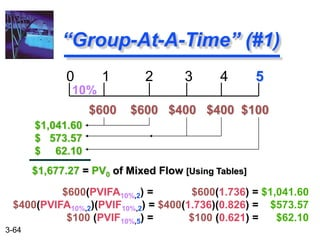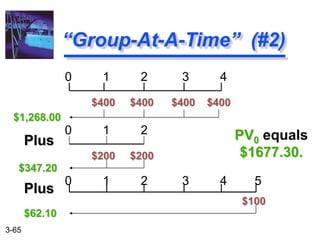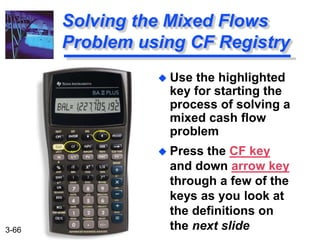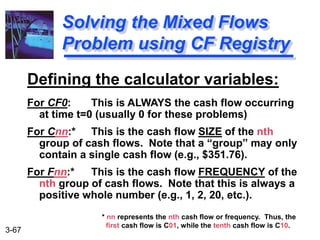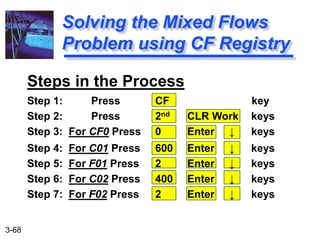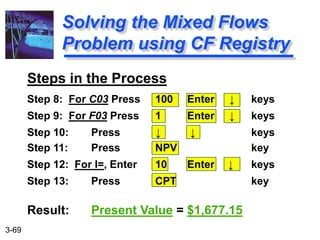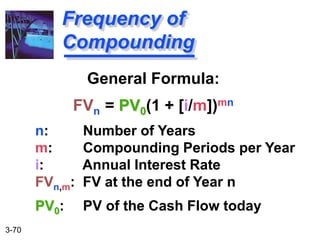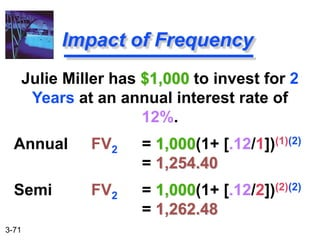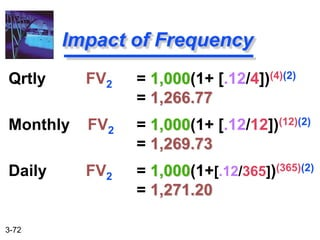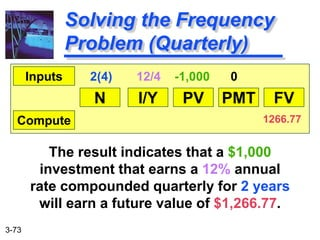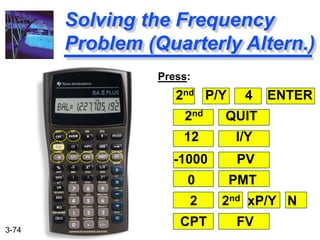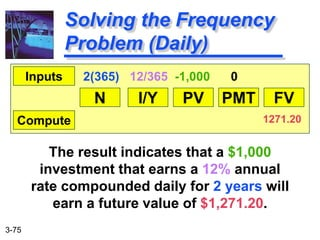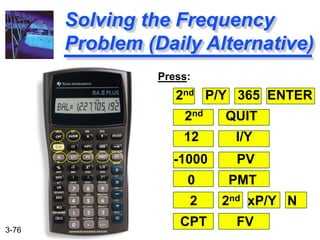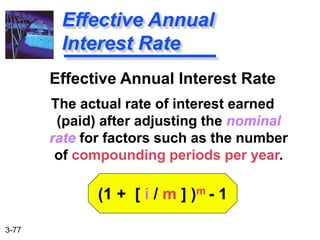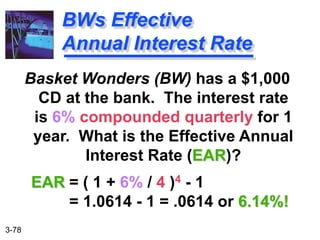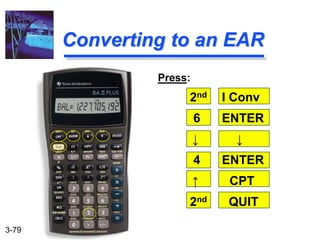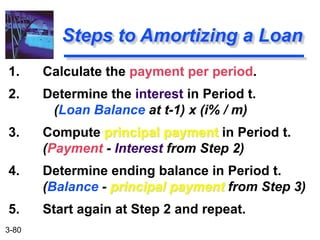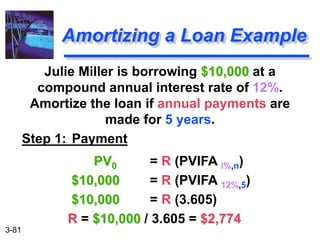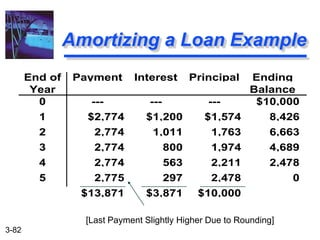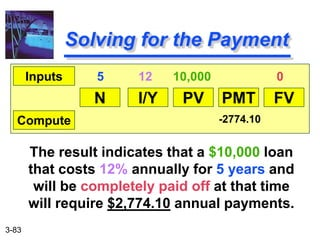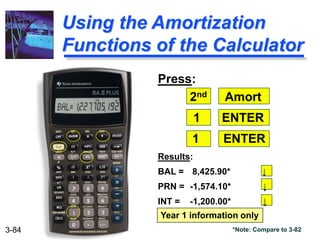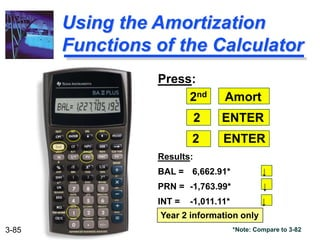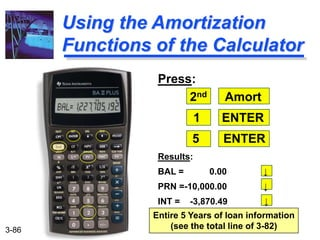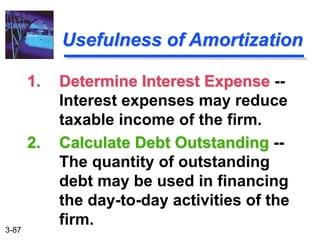1 of 87

### 0273685988_ch03.ppt

1. 3-1 Chapter 3 Time Value of Money © Pearson Education Limited 2004 Fundamentals of Financial Management, 12/e Created by: Gregory A. Kuhlemeyer, Ph.D. Carroll College, Waukesha, WI
2. 3-2 After studying Chapter 3, you should be able to: 1. Understand what is meant by "the time value of money." 2. Understand the relationship between present and future value. 3. Describe how the interest rate can be used to adjust the value of cash flows – both forward and backward – to a single point in time. 4. Calculate both the future and present value of: (a) an amount invested today; (b) a stream of equal cash flows (an annuity); and (c) a stream of mixed cash flows. 5. Distinguish between an “ordinary annuity” and an “annuity due.” 6. Use interest factor tables and understand how they provide a shortcut to calculating present and future values. 7. Use interest factor tables to find an unknown interest rate or growth rate when the number of time periods and future and present values are known. 8. Build an “amortization schedule” for an installment-style loan.
3. 3-3 The Time Value of Money  The Interest Rate  Simple Interest  Compound Interest  Amortizing a Loan  Compounding More Than Once per Year
4. 3-4 Obviously, \$10,000 today. You already recognize that there is TIME VALUE TO MONEY!! The Interest Rate Which would you prefer -- \$10,000 today or \$10,000 in 5 years?
5. 3-5 TIME allows you the opportunity to postpone consumption and earn INTEREST. Why TIME? Why is TIME such an important element in your decision?
6. 3-6 Types of Interest Compound Interest Interest paid (earned) on any previous interest earned, as well as on the principal borrowed (lent). Simple Interest Interest paid (earned) on only the original amount, or principal, borrowed (lent).
7. 3-7 Simple Interest Formula Formula SI = P0(i)(n) SI: Simple Interest P0: Deposit today (t=0) i: Interest Rate per Period n: Number of Time Periods
8. 3-8 SI = P0(i)(n) = \$1,000(.07)(2) = \$140 Simple Interest Example Assume that you deposit \$1,000 in an account earning 7% simple interest for 2 years. What is the accumulated interest at the end of the 2nd year?
9. 3-9 FV = P0 + SI = \$1,000 + \$140 = \$1,140  Future Value is the value at some future time of a present amount of money, or a series of payments, evaluated at a given interest rate. Simple Interest (FV) What is the Future Value (FV) of the deposit?
10. 3-10 The Present Value is simply the \$1,000 you originally deposited. That is the value today!  Present Value is the current value of a future amount of money, or a series of payments, evaluated at a given interest rate. Simple Interest (PV) What is the Present Value (PV) of the previous problem?
11. 3-11 0 5000 10000 15000 20000 1st Year 10th Year 20th Year 30th Year Future Value of a Single \$1,000 Deposit 10% Simple Interest 7% Compound Interest 10% Compound Interest Why Compound Interest? Future Value (U.S. Dollars)
12. 3-12 Assume that you deposit \$1,000 at a compound interest rate of 7% for 2 years. Future Value Single Deposit (Graphic) 0 1 2 \$1,000 FV2 7%
13. 3-13 FV1 = P0 (1+i)1 = \$1,000 (1.07) = \$1,070 Compound Interest You earned \$70 interest on your \$1,000 deposit over the first year. This is the same amount of interest you would earn under simple interest. Future Value Single Deposit (Formula)
14. 3-14 FV1 = P0 (1+i)1 = \$1,000 (1.07) = \$1,070 FV2 = FV1 (1+i)1 = P0 (1+i)(1+i) = \$1,000(1.07)(1.07) = P0 (1+i)2 = \$1,000(1.07)2 = \$1,144.90 You earned an EXTRA \$4.90 in Year 2 with compound over simple interest. Future Value Single Deposit (Formula)
15. 3-15 FV1 = P0(1+i)1 FV2 = P0(1+i)2 General Future Value Formula: FVn = P0 (1+i)n or FVn = P0 (FVIFi,n) -- See Table I General Future Value Formula etc.
16. 3-16 FVIFi,n is found on Table I at the end of the book. Valuation Using Table I Period 6% 7% 8% 1 1.060 1.070 1.080 2 1.124 1.145 1.166 3 1.191 1.225 1.260 4 1.262 1.311 1.360 5 1.338 1.403 1.469
17. 3-17 FV2 = \$1,000 (FVIF7%,2) = \$1,000 (1.145) = \$1,145 [Due to Rounding] Using Future Value Tables Period 6% 7% 8% 1 1.060 1.070 1.080 2 1.124 1.145 1.166 3 1.191 1.225 1.260 4 1.262 1.311 1.360 5 1.338 1.403 1.469
18. 3-18 TVM on the Calculator  Use the highlighted row of keys for solving any of the FV, PV, FVA, PVA, FVAD, and PVAD problems N: Number of periods I/Y:Interest rate per period PV: Present value PMT: Payment per period FV: Future value CLR TVM: Clears all of the inputs into the above TVM keys
19. 3-19 Using The TI BAII+ Calculator N I/Y PV PMT FV Inputs Compute Focus on 3rd Row of keys (will be displayed in slides as shown above)
20. 3-20 Entering the FV Problem Press: 2nd CLR TVM 2 N 7 I/Y -1000 PV 0 PMT CPT FV
21. 3-21 N: 2 Periods (enter as 2) I/Y: 7% interest rate per period (enter as 7 NOT .07) PV: \$1,000 (enter as negative as you have “less”) PMT: Not relevant in this situation (enter as 0) FV: Compute (Resulting answer is positive) Solving the FV Problem N I/Y PV PMT FV Inputs Compute 2 7 -1,000 0 1,144.90
22. 3-22 Julie Miller wants to know how large her deposit of \$10,000 today will become at a compound annual interest rate of 10% for 5 years. Story Problem Example 0 1 2 3 4 5 \$10,000 FV5 10%
23. 3-23 Calculation based on Table I: FV5 = \$10,000 (FVIF10%, 5) = \$10,000 (1.611) = \$16,110 [Due to Rounding] Story Problem Solution  Calculation based on general formula: FVn = P0 (1+i)n FV5 = \$10,000 (1+ 0.10)5 = \$16,105.10
24. 3-24 Entering the FV Problem Press: 2nd CLR TVM 5 N 10 I/Y -10000 PV 0 PMT CPT FV
25. 3-25 The result indicates that a \$10,000 investment that earns 10% annually for 5 years will result in a future value of \$16,105.10. Solving the FV Problem N I/Y PV PMT FV Inputs Compute 5 10 -10,000 0 16,105.10
26. 3-26 We will use the “Rule-of-72”. Double Your Money!!! Quick! How long does it take to double \$5,000 at a compound rate of 12% per year (approx.)?
27. 3-27 Approx. Years to Double = 72 / i% 72 / 12% = 6 Years [Actual Time is 6.12 Years] The “Rule-of-72” Quick! How long does it take to double \$5,000 at a compound rate of 12% per year (approx.)?
28. 3-28 The result indicates that a \$1,000 investment that earns 12% annually will double to \$2,000 in 6.12 years. Note: 72/12% = approx. 6 years Solving the Period Problem N I/Y PV PMT FV Inputs Compute 12 -1,000 0 +2,000 6.12 years
29. 3-29 Assume that you need \$1,000 in 2 years. Let’s examine the process to determine how much you need to deposit today at a discount rate of 7% compounded annually. 0 1 2 \$1,000 7% PV1 PV0 Present Value Single Deposit (Graphic)
30. 3-30 PV0 = FV2 / (1+i)2 = \$1,000 / (1.07)2 = FV2 / (1+i)2 = \$873.44 Present Value Single Deposit (Formula) 0 1 2 \$1,000 7% PV0
31. 3-31 PV0 = FV1 / (1+i)1 PV0 = FV2 / (1+i)2 General Present Value Formula: PV0 = FVn / (1+i)n or PV0 = FVn (PVIFi,n) -- See Table II General Present Value Formula etc.
32. 3-32 PVIFi,n is found on Table II at the end of the book. Valuation Using Table II Period 6% 7% 8% 1 .943 .935 .926 2 .890 .873 .857 3 .840 .816 .794 4 .792 .763 .735 5 .747 .713 .681
33. 3-33 PV2 = \$1,000 (PVIF7%,2) = \$1,000 (.873) = \$873 [Due to Rounding] Using Present Value Tables Period 6% 7% 8% 1 .943 .935 .926 2 .890 .873 .857 3 .840 .816 .794 4 .792 .763 .735 5 .747 .713 .681
34. 3-34 N: 2 Periods (enter as 2) I/Y: 7% interest rate per period (enter as 7 NOT .07) PV: Compute (Resulting answer is negative “deposit”) PMT: Not relevant in this situation (enter as 0) FV: \$1,000 (enter as positive as you “receive \$”) Solving the PV Problem N I/Y PV PMT FV Inputs Compute 2 7 0 +1,000 -873.44
35. 3-35 Julie Miller wants to know how large of a deposit to make so that the money will grow to \$10,000 in 5 years at a discount rate of 10%. Story Problem Example 0 1 2 3 4 5 \$10,000 PV0 10%
36. 3-36  Calculation based on general formula: PV0 = FVn / (1+i)n PV0 = \$10,000 / (1+ 0.10)5 = \$6,209.21  Calculation based on Table I: PV0 = \$10,000 (PVIF10%, 5) = \$10,000 (.621) = \$6,210.00 [Due to Rounding] Story Problem Solution
37. 3-37 Solving the PV Problem N I/Y PV PMT FV Inputs Compute 5 10 0 +10,000 -6,209.21 The result indicates that a \$10,000 future value that will earn 10% annually for 5 years requires a \$6,209.21 deposit today (present value).
38. 3-38 Types of Annuities  Ordinary Annuity: Payments or receipts occur at the end of each period.  Annuity Due: Payments or receipts occur at the beginning of each period.  An Annuity represents a series of equal payments (or receipts) occurring over a specified number of equidistant periods.
39. 3-39 Examples of Annuities  Student Loan Payments  Car Loan Payments  Insurance Premiums  Mortgage Payments  Retirement Savings
40. 3-40 Parts of an Annuity 0 1 2 3 \$100 \$100 \$100 (Ordinary Annuity) End of Period 1 End of Period 2 Today Equal Cash Flows Each 1 Period Apart End of Period 3
41. 3-41 Parts of an Annuity 0 1 2 3 \$100 \$100 \$100 (Annuity Due) Beginning of Period 1 Beginning of Period 2 Today Equal Cash Flows Each 1 Period Apart Beginning of Period 3
42. 3-42 FVAn = R(1+i)n-1 + R(1+i)n-2 + ... + R(1+i)1 + R(1+i)0 Overview of an Ordinary Annuity -- FVA R R R 0 1 2 n n+1 FVAn R = Periodic Cash Flow Cash flows occur at the end of the period i% . . .
43. 3-43 FVA3 = \$1,000(1.07)2 + \$1,000(1.07)1 + \$1,000(1.07)0 = \$1,145 + \$1,070 + \$1,000 = \$3,215 Example of an Ordinary Annuity -- FVA \$1,000 \$1,000 \$1,000 0 1 2 3 4 \$3,215 = FVA3 7% \$1,070 \$1,145 Cash flows occur at the end of the period
44. 3-44 Hint on Annuity Valuation The future value of an ordinary annuity can be viewed as occurring at the end of the last cash flow period, whereas the future value of an annuity due can be viewed as occurring at the beginning of the last cash flow period.
45. 3-45 FVAn = R (FVIFAi%,n) FVA3 = \$1,000 (FVIFA7%,3) = \$1,000 (3.215) = \$3,215 Valuation Using Table III Period 6% 7% 8% 1 1.000 1.000 1.000 2 2.060 2.070 2.080 3 3.184 3.215 3.246 4 4.375 4.440 4.506 5 5.637 5.751 5.867
46. 3-46 N: 3 Periods (enter as 3 year-end deposits) I/Y: 7% interest rate per period (enter as 7 NOT .07) PV: Not relevant in this situation (no beg value) PMT: \$1,000 (negative as you deposit annually) FV: Compute (Resulting answer is positive) Solving the FVA Problem N I/Y PV PMT FV Inputs Compute 3 7 0 -1,000 3,214.90
47. 3-47 FVADn = R(1+i)n + R(1+i)n-1 + ... + R(1+i)2 + R(1+i)1 = FVAn (1+i) Overview View of an Annuity Due -- FVAD R R R R R 0 1 2 3 n-1 n FVADn i% . . . Cash flows occur at the beginning of the period
48. 3-48 FVAD3 = \$1,000(1.07)3 + \$1,000(1.07)2 + \$1,000(1.07)1 = \$1,225 + \$1,145 + \$1,070 = \$3,440 Example of an Annuity Due -- FVAD \$1,000 \$1,000 \$1,000 \$1,070 0 1 2 3 4 \$3,440 = FVAD3 7% \$1,225 \$1,145 Cash flows occur at the beginning of the period
49. 3-49 FVADn = R (FVIFAi%,n)(1+i) FVAD3 = \$1,000 (FVIFA7%,3)(1.07) = \$1,000 (3.215)(1.07) = \$3,440 Valuation Using Table III Period 6% 7% 8% 1 1.000 1.000 1.000 2 2.060 2.070 2.080 3 3.184 3.215 3.246 4 4.375 4.440 4.506 5 5.637 5.751 5.867
50. 3-50 Solving the FVAD Problem N I/Y PV PMT FV Inputs Compute 3 7 0 -1,000 3,439.94 Complete the problem the same as an “ordinary annuity” problem, except you must change the calculator setting to “BGN” first. Don’t forget to change back! Step 1: Press 2nd BGN keys Step 2: Press 2nd SET keys Step 3: Press 2nd QUIT keys
51. 3-51 PVAn = R/(1+i)1 + R/(1+i)2 + ... + R/(1+i)n Overview of an Ordinary Annuity -- PVA R R R 0 1 2 n n+1 PVAn R = Periodic Cash Flow i% . . . Cash flows occur at the end of the period
52. 3-52 PVA3 = \$1,000/(1.07)1 + \$1,000/(1.07)2 + \$1,000/(1.07)3 = \$934.58 + \$873.44 + \$816.30 = \$2,624.32 Example of an Ordinary Annuity -- PVA \$1,000 \$1,000 \$1,000 0 1 2 3 4 \$2,624.32 = PVA3 7% \$934.58 \$873.44 \$816.30 Cash flows occur at the end of the period
53. 3-53 Hint on Annuity Valuation The present value of an ordinary annuity can be viewed as occurring at the beginning of the first cash flow period, whereas the future value of an annuity due can be viewed as occurring at the end of the first cash flow period.
54. 3-54 PVAn = R (PVIFAi%,n) PVA3 = \$1,000 (PVIFA7%,3) = \$1,000 (2.624) = \$2,624 Valuation Using Table IV Period 6% 7% 8% 1 0.943 0.935 0.926 2 1.833 1.808 1.783 3 2.673 2.624 2.577 4 3.465 3.387 3.312 5 4.212 4.100 3.993
55. 3-55 N: 3 Periods (enter as 3 year-end deposits) I/Y: 7% interest rate per period (enter as 7 NOT .07) PV: Compute (Resulting answer is positive) PMT: \$1,000 (negative as you deposit annually) FV: Not relevant in this situation (no ending value) Solving the PVA Problem N I/Y PV PMT FV Inputs Compute 3 7 -1,000 0 2,624.32
56. 3-56 PVADn = R/(1+i)0 + R/(1+i)1 + ... + R/(1+i)n-1 = PVAn (1+i) Overview of an Annuity Due -- PVAD R R R R 0 1 2 n-1 n PVADn R: Periodic Cash Flow i% . . . Cash flows occur at the beginning of the period
57. 3-57 PVADn = \$1,000/(1.07)0 + \$1,000/(1.07)1 + \$1,000/(1.07)2 = \$2,808.02 Example of an Annuity Due -- PVAD \$1,000.00 \$1,000 \$1,000 0 1 2 3 4 \$2,808.02 = PVADn 7% \$ 934.58 \$ 873.44 Cash flows occur at the beginning of the period
58. 3-58 PVADn = R (PVIFAi%,n)(1+i) PVAD3 = \$1,000 (PVIFA7%,3)(1.07) = \$1,000 (2.624)(1.07) = \$2,808 Valuation Using Table IV Period 6% 7% 8% 1 0.943 0.935 0.926 2 1.833 1.808 1.783 3 2.673 2.624 2.577 4 3.465 3.387 3.312 5 4.212 4.100 3.993
59. 3-59 Solving the PVAD Problem N I/Y PV PMT FV Inputs Compute 3 7 -1,000 0 2,808.02 Complete the problem the same as an “ordinary annuity” problem, except you must change the calculator setting to “BGN” first. Don’t forget to change back! Step 1: Press 2nd BGN keys Step 2: Press 2nd SET keys Step 3: Press 2nd QUIT keys
60. 3-60 1. Read problem thoroughly 2. Create a time line 3. Put cash flows and arrows on time line 4. Determine if it is a PV or FV problem 5. Determine if solution involves a single CF, annuity stream(s), or mixed flow 6. Solve the problem 7. Check with financial calculator (optional) Steps to Solve Time Value of Money Problems
61. 3-61 Julie Miller will receive the set of cash flows below. What is the Present Value at a discount rate of 10%. Mixed Flows Example 0 1 2 3 4 5 \$600 \$600 \$400 \$400 \$100 PV0 10%
62. 3-62 1. Solve a “piece-at-a-time” by discounting each piece back to t=0. 2. Solve a “group-at-a-time” by first breaking problem into groups of annuity streams and any single cash flow groups. Then discount each group back to t=0. How to Solve?
63. 3-63 “Piece-At-A-Time” 0 1 2 3 4 5 \$600 \$600 \$400 \$400 \$100 10% \$545.45 \$495.87 \$300.53 \$273.21 \$ 62.09 \$1677.15 = PV0 of the Mixed Flow
64. 3-64 “Group-At-A-Time” (#1) 0 1 2 3 4 5 \$600 \$600 \$400 \$400 \$100 10% \$1,041.60 \$ 573.57 \$ 62.10 \$1,677.27 = PV0 of Mixed Flow [Using Tables] \$600(PVIFA10%,2) = \$600(1.736) = \$1,041.60 \$400(PVIFA10%,2)(PVIF10%,2) = \$400(1.736)(0.826) = \$573.57 \$100 (PVIF10%,5) = \$100 (0.621) = \$62.10
65. 3-65 “Group-At-A-Time” (#2) 0 1 2 3 4 \$400 \$400 \$400 \$400 PV0 equals \$1677.30. 0 1 2 \$200 \$200 0 1 2 3 4 5 \$100 \$1,268.00 \$347.20 \$62.10 Plus Plus
66. 3-66  Use the highlighted key for starting the process of solving a mixed cash flow problem  Press the CF key and down arrow key through a few of the keys as you look at the definitions on the next slide Solving the Mixed Flows Problem using CF Registry
67. 3-67 Defining the calculator variables: For CF0: This is ALWAYS the cash flow occurring at time t=0 (usually 0 for these problems) For Cnn:* This is the cash flow SIZE of the nth group of cash flows. Note that a “group” may only contain a single cash flow (e.g., \$351.76). For Fnn:* This is the cash flow FREQUENCY of the nth group of cash flows. Note that this is always a positive whole number (e.g., 1, 2, 20, etc.). Solving the Mixed Flows Problem using CF Registry * nn represents the nth cash flow or frequency. Thus, the first cash flow is C01, while the tenth cash flow is C10.
68. 3-68 Solving the Mixed Flows Problem using CF Registry Steps in the Process Step 1: Press CF key Step 2: Press 2nd CLR Work keys Step 3: For CF0 Press 0 Enter ↓ keys Step 4: For C01 Press 600 Enter ↓ keys Step 5: For F01 Press 2 Enter ↓ keys Step 6: For C02 Press 400 Enter ↓ keys Step 7: For F02 Press 2 Enter ↓ keys
69. 3-69 Solving the Mixed Flows Problem using CF Registry Steps in the Process Step 8: For C03 Press 100 Enter ↓ keys Step 9: For F03 Press 1 Enter ↓ keys Step 10: Press ↓ ↓ keys Step 11: Press NPV key Step 12: For I=, Enter 10 Enter ↓ keys Step 13: Press CPT key Result: Present Value = \$1,677.15
70. 3-70 General Formula: FVn = PV0(1 + [i/m])mn n: Number of Years m: Compounding Periods per Year i: Annual Interest Rate FVn,m: FV at the end of Year n PV0: PV of the Cash Flow today Frequency of Compounding
71. 3-71 Julie Miller has \$1,000 to invest for 2 Years at an annual interest rate of 12%. Annual FV2 = 1,000(1+ [.12/1])(1)(2) = 1,254.40 Semi FV2 = 1,000(1+ [.12/2])(2)(2) = 1,262.48 Impact of Frequency
72. 3-72 Qrtly FV2 = 1,000(1+ [.12/4])(4)(2) = 1,266.77 Monthly FV2 = 1,000(1+ [.12/12])(12)(2) = 1,269.73 Daily FV2 = 1,000(1+[.12/365])(365)(2) = 1,271.20 Impact of Frequency
73. 3-73 The result indicates that a \$1,000 investment that earns a 12% annual rate compounded quarterly for 2 years will earn a future value of \$1,266.77. Solving the Frequency Problem (Quarterly) N I/Y PV PMT FV Inputs Compute 2(4) 12/4 -1,000 0 1266.77
74. 3-74 Solving the Frequency Problem (Quarterly Altern.) Press: 2nd P/Y 4 ENTER 2nd QUIT 12 I/Y -1000 PV 0 PMT 2 2nd xP/Y N CPT FV
75. 3-75 The result indicates that a \$1,000 investment that earns a 12% annual rate compounded daily for 2 years will earn a future value of \$1,271.20. Solving the Frequency Problem (Daily) N I/Y PV PMT FV Inputs Compute 2(365) 12/365 -1,000 0 1271.20
76. 3-76 Solving the Frequency Problem (Daily Alternative) Press: 2nd P/Y 365 ENTER 2nd QUIT 12 I/Y -1000 PV 0 PMT 2 2nd xP/Y N CPT FV
77. 3-77 Effective Annual Interest Rate The actual rate of interest earned (paid) after adjusting the nominal rate for factors such as the number of compounding periods per year. (1 + [ i / m ] )m - 1 Effective Annual Interest Rate
78. 3-78 Basket Wonders (BW) has a \$1,000 CD at the bank. The interest rate is 6% compounded quarterly for 1 year. What is the Effective Annual Interest Rate (EAR)? EAR = ( 1 + 6% / 4 )4 - 1 = 1.0614 - 1 = .0614 or 6.14%! BWs Effective Annual Interest Rate
79. 3-79 Converting to an EAR Press: 2nd I Conv 6 ENTER ↓ ↓ 4 ENTER ↑ CPT 2nd QUIT
80. 3-80 1. Calculate the payment per period. 2. Determine the interest in Period t. (Loan Balance at t-1) x (i% / m) 3. Compute principal payment in Period t. (Payment - Interest from Step 2) 4. Determine ending balance in Period t. (Balance - principal payment from Step 3) 5. Start again at Step 2 and repeat. Steps to Amortizing a Loan
81. 3-81 Julie Miller is borrowing \$10,000 at a compound annual interest rate of 12%. Amortize the loan if annual payments are made for 5 years. Step 1: Payment PV0 = R (PVIFA i%,n) \$10,000 = R (PVIFA 12%,5) \$10,000 = R (3.605) R = \$10,000 / 3.605 = \$2,774 Amortizing a Loan Example
82. 3-82 Amortizing a Loan Example End of Year Payment Interest Principal Ending Balance 0 --- --- --- \$10,000 1 \$2,774 \$1,200 \$1,574 8,426 2 2,774 1,011 1,763 6,663 3 2,774 800 1,974 4,689 4 2,774 563 2,211 2,478 5 2,775 297 2,478 0 \$13,871 \$3,871 \$10,000 [Last Payment Slightly Higher Due to Rounding]
83. 3-83 The result indicates that a \$10,000 loan that costs 12% annually for 5 years and will be completely paid off at that time will require \$2,774.10 annual payments. Solving for the Payment N I/Y PV PMT FV Inputs Compute 5 12 10,000 0 -2774.10
84. 3-84 Using the Amortization Functions of the Calculator Press: 2nd Amort 1 ENTER 1 ENTER Results: BAL = 8,425.90* ↓ PRN = -1,574.10* ↓ INT = -1,200.00* ↓ Year 1 information only *Note: Compare to 3-82
85. 3-85 Using the Amortization Functions of the Calculator Press: 2nd Amort 2 ENTER 2 ENTER Results: BAL = 6,662.91* ↓ PRN = -1,763.99* ↓ INT = -1,011.11* ↓ Year 2 information only *Note: Compare to 3-82
86. 3-86 Using the Amortization Functions of the Calculator Press: 2nd Amort 1 ENTER 5 ENTER Results: BAL = 0.00 ↓ PRN =-10,000.00 ↓ INT = -3,870.49 ↓ Entire 5 Years of loan information (see the total line of 3-82)
87. 3-87 Usefulness of Amortization 2. Calculate Debt Outstanding -- The quantity of outstanding debt may be used in financing the day-to-day activities of the firm. 1. Determine Interest Expense -- Interest expenses may reduce taxable income of the firm.